# Subdivision Curves¶

$\newcommand{\dotp}{\langle #1, #2 \rangle}$ $\newcommand{\enscond}{\lbrace #1, #2 \rbrace}$ $\newcommand{\pd}{ \frac{ \partial #1}{\partial #2} }$ $\newcommand{\umin}{\underset{#1}{\min}\;}$ $\newcommand{\umax}{\underset{#1}{\max}\;}$ $\newcommand{\umin}{\underset{#1}{\min}\;}$ $\newcommand{\uargmin}{\underset{#1}{argmin}\;}$ $\newcommand{\norm}{\|#1\|}$ $\newcommand{\abs}{\left|#1\right|}$ $\newcommand{\choice}{ \left\{ \begin{array}{l} #1 \end{array} \right. }$ $\newcommand{\pa}{\left(#1\right)}$ $\newcommand{\diag}{{diag}\left( #1 \right)}$ $\newcommand{\qandq}{\quad\text{and}\quad}$ $\newcommand{\qwhereq}{\quad\text{where}\quad}$ $\newcommand{\qifq}{ \quad \text{if} \quad }$ $\newcommand{\qarrq}{ \quad \Longrightarrow \quad }$ $\newcommand{\ZZ}{\mathbb{Z}}$ $\newcommand{\CC}{\mathbb{C}}$ $\newcommand{\RR}{\mathbb{R}}$ $\newcommand{\EE}{\mathbb{E}}$ $\newcommand{\Zz}{\mathcal{Z}}$ $\newcommand{\Ww}{\mathcal{W}}$ $\newcommand{\Vv}{\mathcal{V}}$ $\newcommand{\Nn}{\mathcal{N}}$ $\newcommand{\NN}{\mathcal{N}}$ $\newcommand{\Hh}{\mathcal{H}}$ $\newcommand{\Bb}{\mathcal{B}}$ $\newcommand{\Ee}{\mathcal{E}}$ $\newcommand{\Cc}{\mathcal{C}}$ $\newcommand{\Gg}{\mathcal{G}}$ $\newcommand{\Ss}{\mathcal{S}}$ $\newcommand{\Pp}{\mathcal{P}}$ $\newcommand{\Ff}{\mathcal{F}}$ $\newcommand{\Xx}{\mathcal{X}}$ $\newcommand{\Mm}{\mathcal{M}}$ $\newcommand{\Ii}{\mathcal{I}}$ $\newcommand{\Dd}{\mathcal{D}}$ $\newcommand{\Ll}{\mathcal{L}}$ $\newcommand{\Tt}{\mathcal{T}}$ $\newcommand{\si}{\sigma}$ $\newcommand{\al}{\alpha}$ $\newcommand{\la}{\lambda}$ $\newcommand{\ga}{\gamma}$ $\newcommand{\Ga}{\Gamma}$ $\newcommand{\La}{\Lambda}$ $\newcommand{\si}{\sigma}$ $\newcommand{\Si}{\Sigma}$ $\newcommand{\be}{\beta}$ $\newcommand{\de}{\delta}$ $\newcommand{\De}{\Delta}$ $\newcommand{\phi}{\varphi}$ $\newcommand{\th}{\theta}$ $\newcommand{\om}{\omega}$ $\newcommand{\Om}{\Omega}$

Subdvision methods progressively refine a discrete curve and converge to a smooth curve. This allows to perform an interpolation or approximation of a given coarse dataset.

In :
addpath('toolbox_signal')


## Curve Subdivision ¶

Starting from an initial set of control points (which can be seen as a coarse curve), subdivision produces a smooth 2-D curve.

Shortcut to plot a periodic curve.

In :
ms = 20; lw = 1.5;
myplot = @(f,c)plot(f([1:end 1]), c, 'LineWidth', lw, 'MarkerSize', ms);
myaxis = @(rho)axis([-rho 1+rho -rho 1+rho], 'off');


We represent a dicretized curve of $N$ points as a vector of complex numbers $f \in \CC^N$. Since we consider periodic boundary conditions, we assume the vectors have periodic boundary conditions.

Define the initial coarse set of control points $f_0 \in \CC^{N_0}$.

In :
f0 =    [0.11 0.18 0.26 0.36 0.59 0.64 0.80 0.89 0.58 0.22 0.18 0.30 0.58 0.43 0.42]' + ...
1i * [0.91 0.55 0.91 0.58 0.78 0.51 0.81 0.56 0.10 0.16 0.35 0.42 0.40 0.24 0.31]';


Rescale it to fit in a box.

In :
f0 = rescale(real(f0),.01,.99) + 1i * rescale(imag(f0),.01,.99);


Display it.

In :
clf; myplot(f0, 'k.-');
myaxis(0);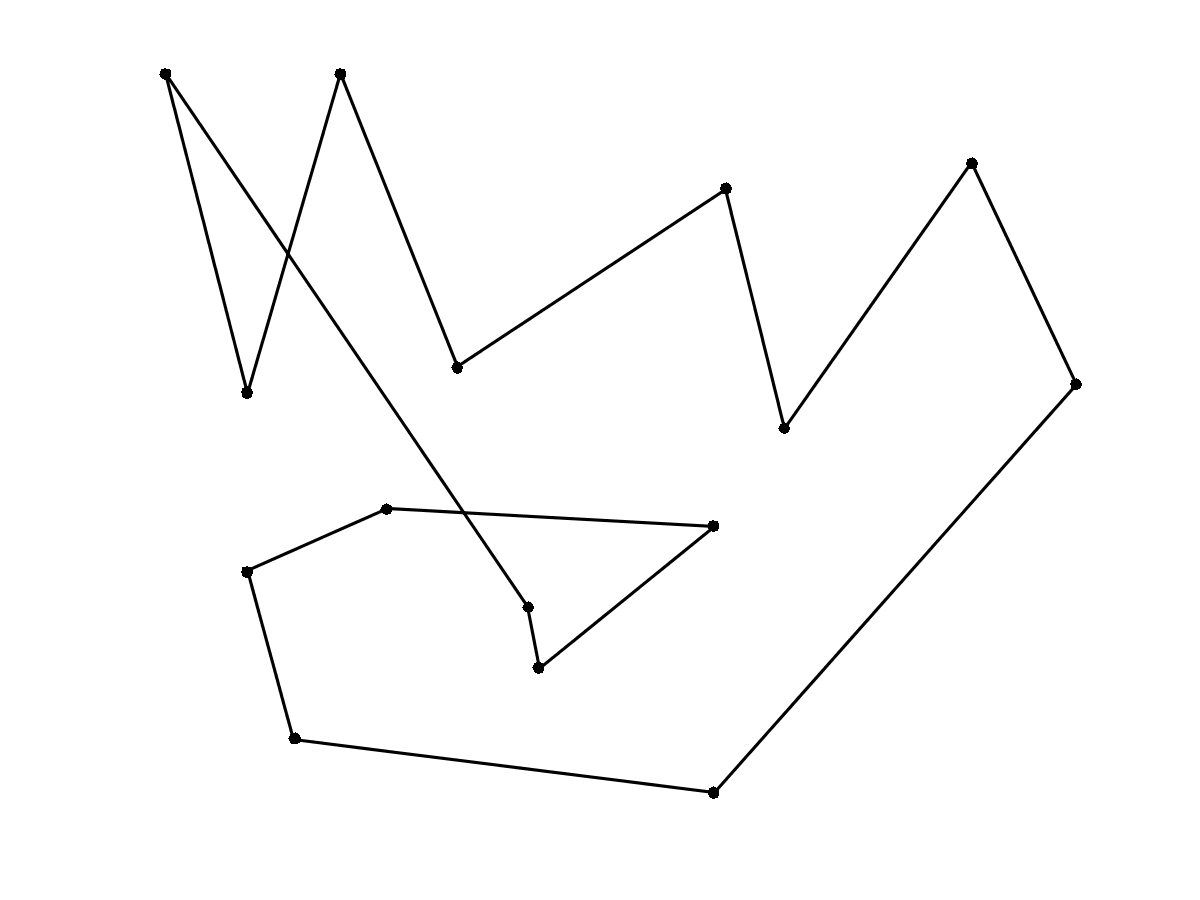One subdivision step reads $$f_{j+1} = (f_j \uparrow 2) \star h.$$ This produces discrete curves $f_j \in \CC^{N_j}$ where $N_j = N_0 2^j$.

Here $\uparrow 2$ is the up-sampling operator $$(f \uparrow 2)_{2i}=f_i \qandq (f \uparrow 2)_{2i+1} = 0.$$

Recall that the periodic discrete convolution is defined as $$(f \star h)_i = \sum_j f_j h_{i-j},$$ where the filter $h$ is zero-padded to reach the same size as $f$.

The low pass filter (subdivision kernel) $h \in \CC^K$ should satisfies $$\sum_i h_i = 2 .$$ This ensure that the center of gravity of the curve stays constant $$\frac{1}{N_j} \sum_{i=1}^{N_j} f_{j,i} = \frac{1}{N_0} \sum_{i=1}^{N_0} f_{0,i}.$$

Define the subdivision operator that maps $f_j$ to $f_{j+1}$.

In :
subdivide = @(f,h)cconvol( upsampling(f), h);


We use here the kernel $$h = \frac{1}{8}[1, 4, 6, 4, 1].$$ It produced a cubic B-spline interpolation.

In :
h = [1 4 6 4 1];
h = 2*h/sum(h(:));


Initilize the subdivision with $f_0$ at scale $j=0$.

In :
f = f0;


Perform one step.

In :
f = subdivide(f,h);


Display the original and filtered discrete curves.

In :
clf; hold on;
myplot(f, 'k.-');
myplot(f0, 'r.--');
myaxis(0);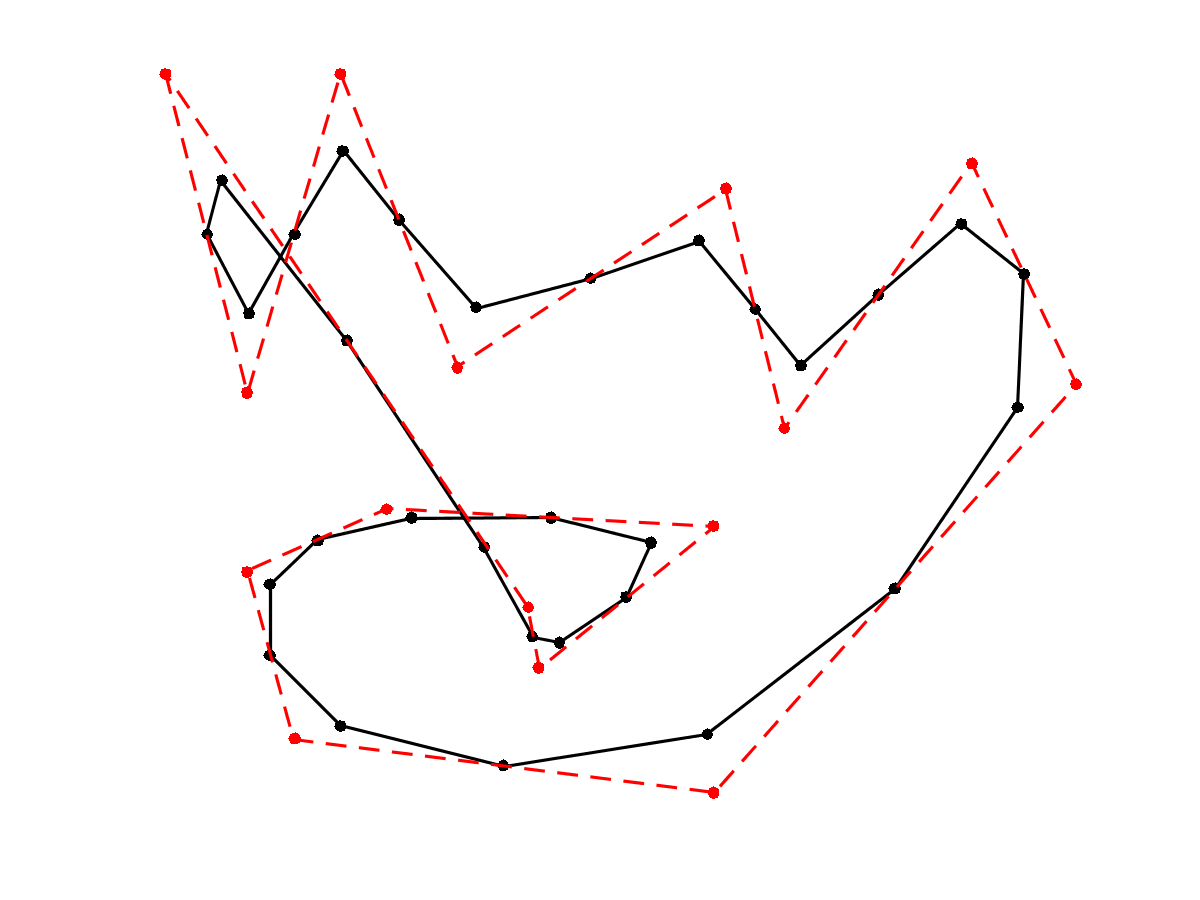Exercise 1

Perform several step of subdivision. Display the different curves.

In :
exo1()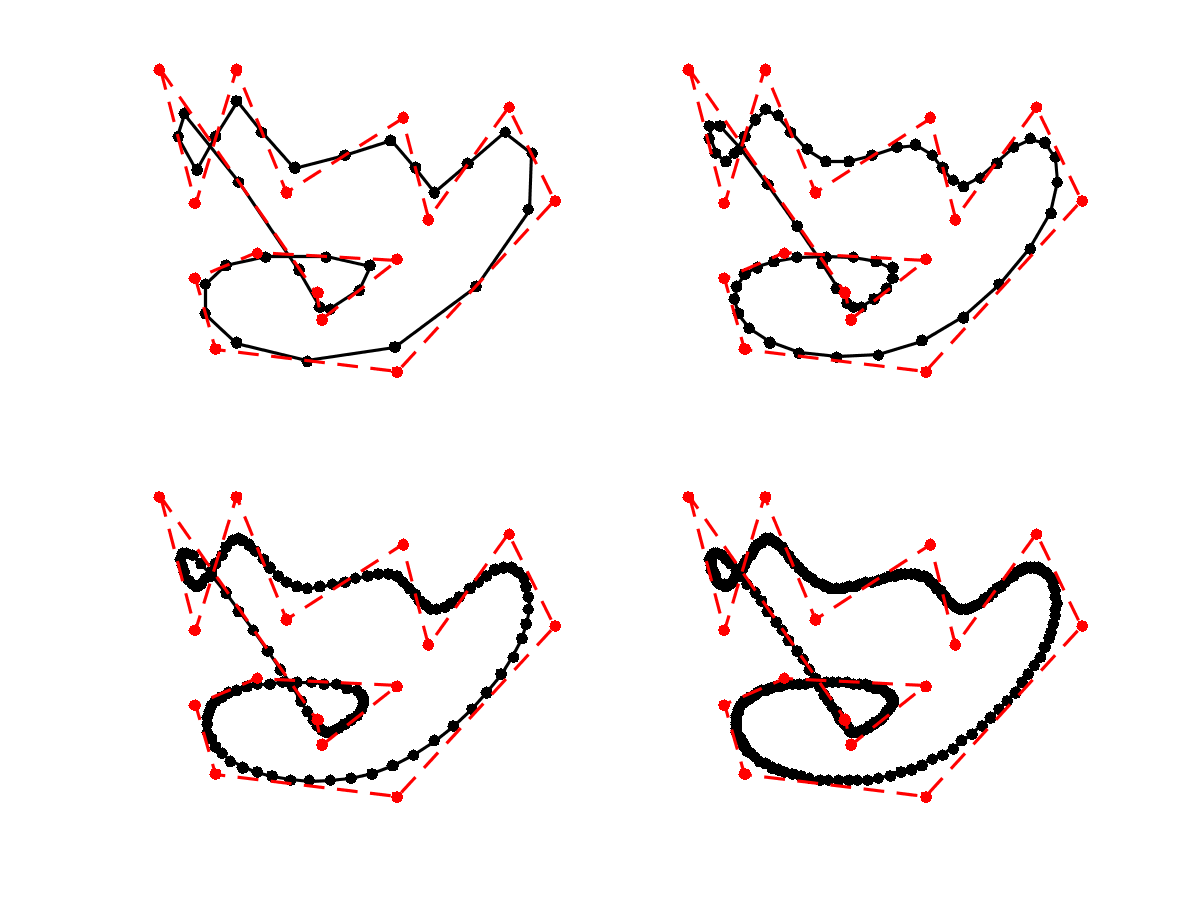In :
%% Insert your code here.


Under some restriction on the kernel $h$, one can show that these discrete curves converges (e.g. in Hausdorff distance) toward a smooth limit curve $f^\star : [0,1] \rightarrow \CC$.

We do not details here sufficient condition to ensure convergence and smoothness of the limitting curve. The interested reader can have a look at DynLevin02 for a review of theoritical guarantees for subdivision.

The limit curve $f^\star$ is a weighted average of the initial points $f_0 = (f_{0,i})_{i=0}^{N_0-1} \in \CC^{N_0}$ using a continuous scaling function $\phi : [0,1] \rightarrow \RR$ $$f^\star(t) = \sum_{i=0}^{N_0-1} f_{0,i} \phi(t-i/N_0).$$ The continuous kernel $\phi$ is a low-pass function which as a compact support of width $K/N_0$. The control point $f_{0,i}$ thus only influences the final curve $f^\star$ around $t=i/N_0$.

The scaling function $\phi$ can be computed as the limit of the sub-division process $f_j$ when starting from $f_0 = \delta = [1,0,\ldots,0]$, which is the Dirac vector.

Exercise 2

Compute the scaling function $\phi$ associated to the subdivision.

In :
exo2()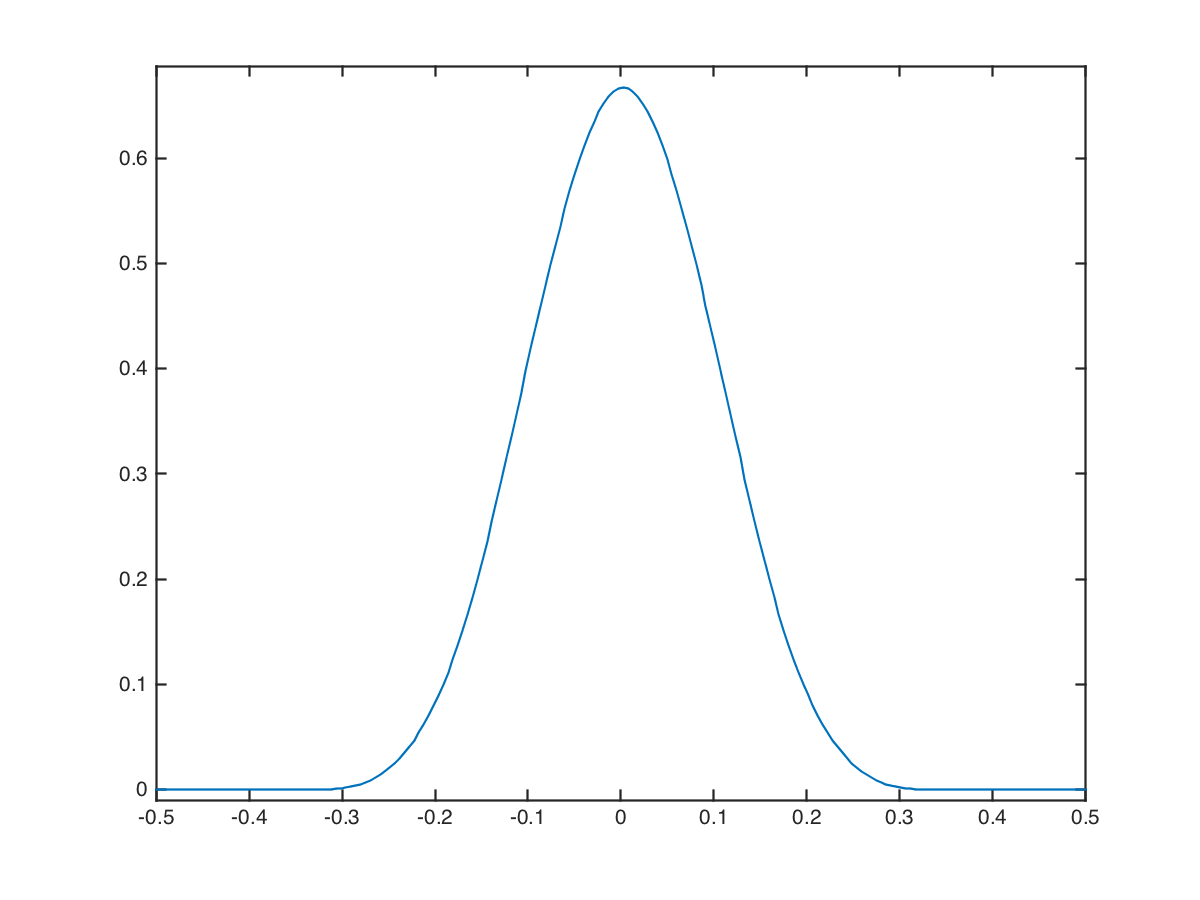In :
%% Insert your code here.


Exercise 3

Test with different configurations of control points.

In :
exo3()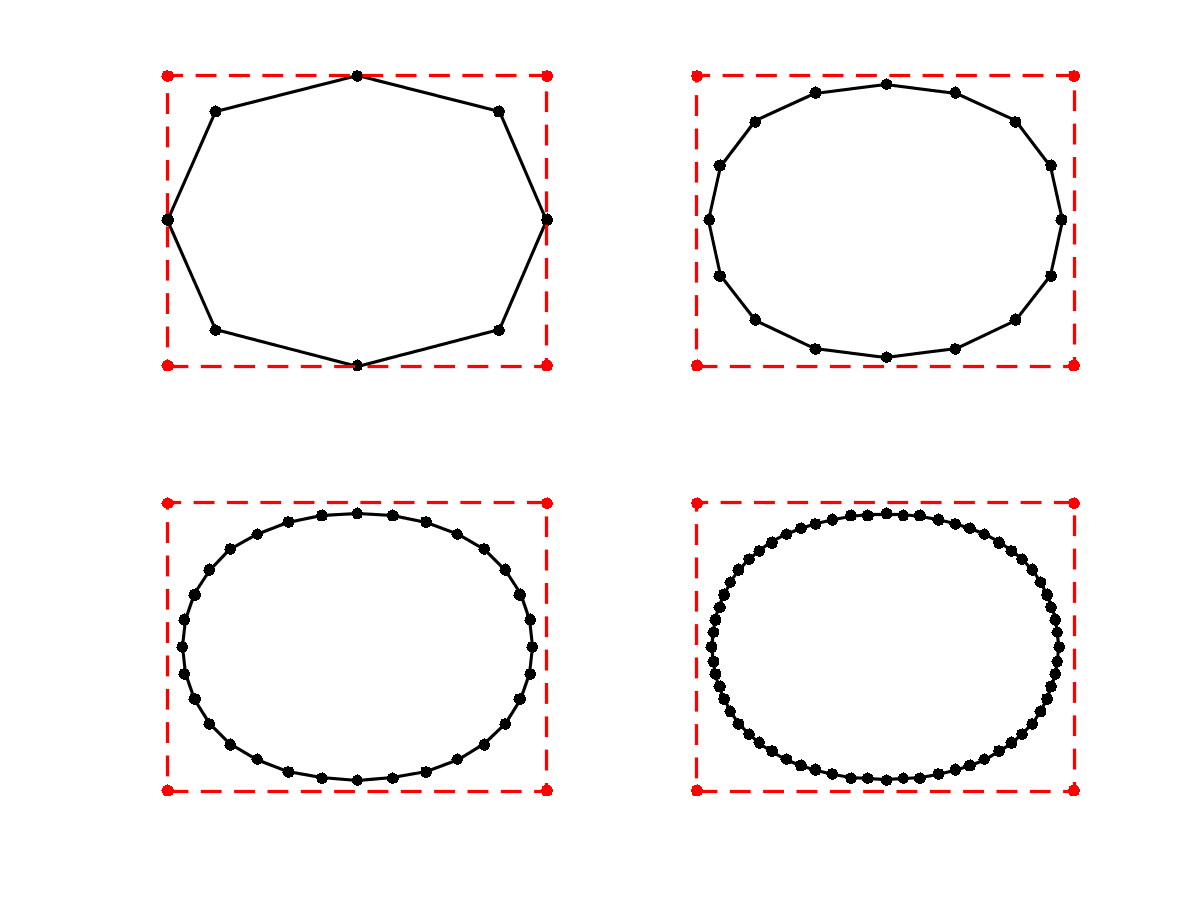In :
%% Insert your code here.


We consider here the Chaikin "corner cutting" scheme Chaikin74.

For a weight $w>1$, it corresponds to the following kernel: $$h = \frac{1}{1+w}[1, w, w, 1].$$ The weight is a tension parameter that controls the properties of the interpolation.

In :
hcc = @(w)[1 w w 1]/(1+w);


For $w=3$, the scaling function $\phi$ is a quadratic B-spline.

Exercise 4

Test the corner-cutting for $w=3$.

In :
exo4()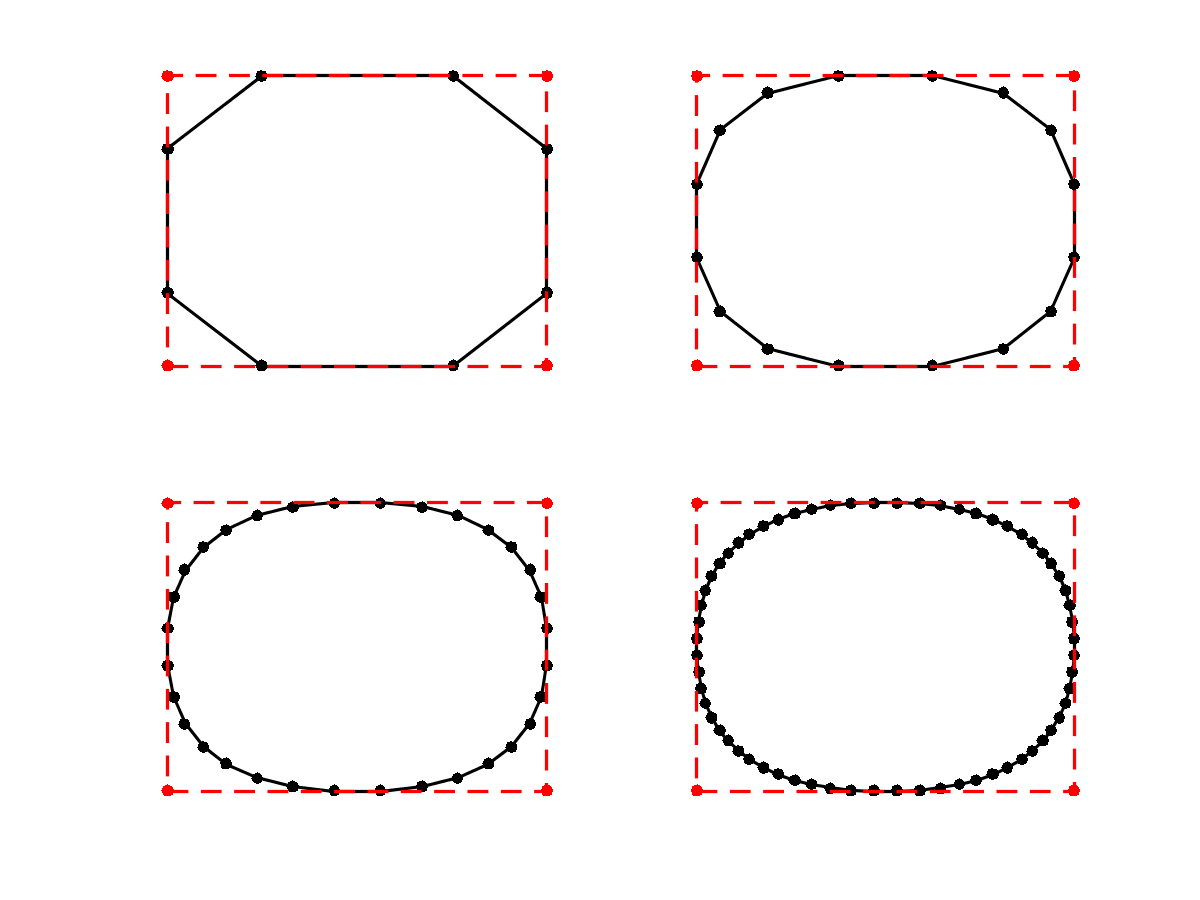In :
%% Insert your code here.


Exercise 5

Test the corner-cutting for vaious values of $w$.

In :
exo5()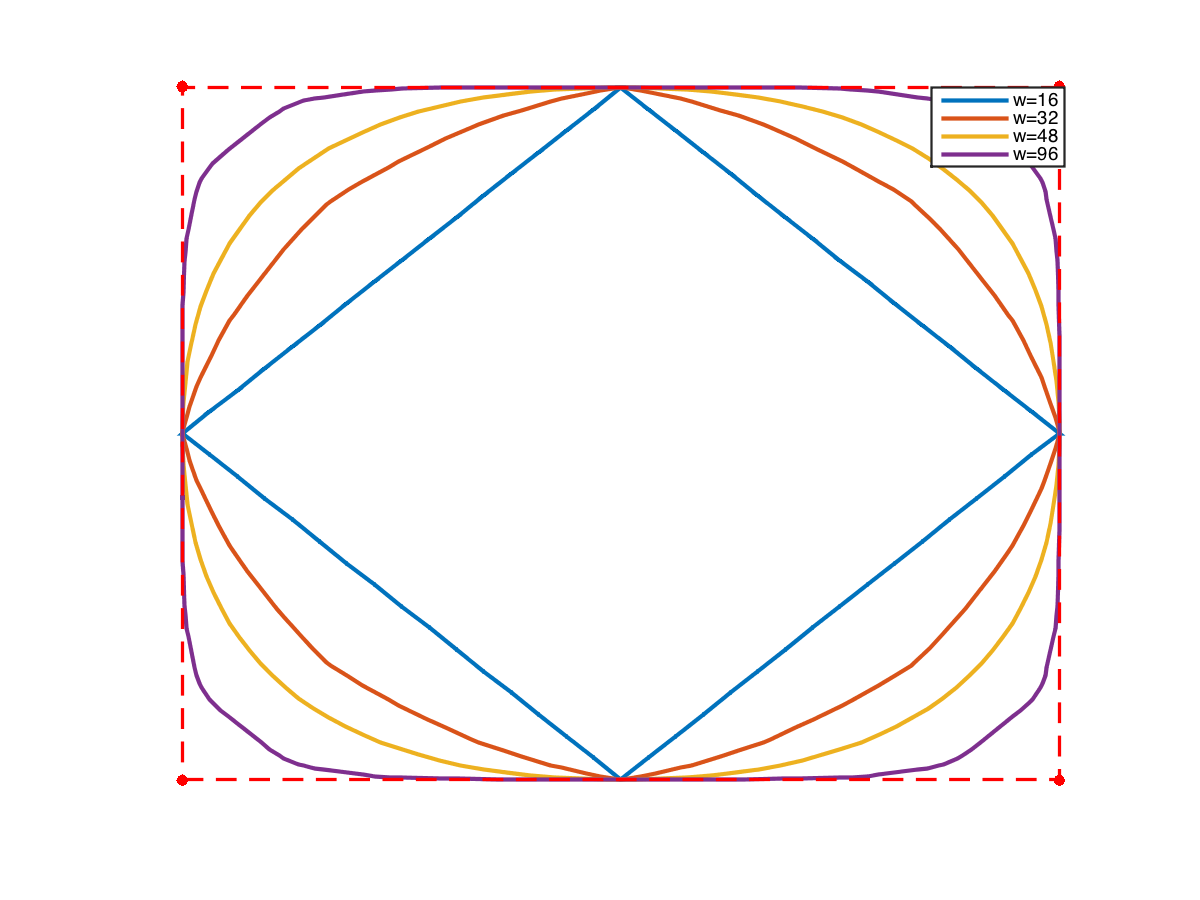In :
%% Insert your code here.


## Interpolating Subdivision¶

Interpolating schemes keeps unchange the set of point at the previous level, and only smooth the position of the added points.

A subdivision is interpolating if the kernel satisfies $$h(0)=1 \qandq \forall i \neq 0, \quad h(2i)=0.$$

We consider the four-point interpolation kernel proposed in DynLevGre87: $$h = [-w, 0, 1/2+w, 1, 1/2+w, -w]$$ where $w>0$ is a tension parameter.

In :
h4pt = @(w)[-w, 0, 1/2+w, 1, 1/2+w, 0, -w];


One usually choose $w=1/16$ wich corresponds to cubic B-spline interpolation. It can be shown to produce $C^1$ curves for $w \in [0, (\sqrt{5}-1)/8 \approx 0.154]$, see DynGreLev91.

Exercise 6

Perform the interpolating subdivision for $w=1/16$.

In :
exo6()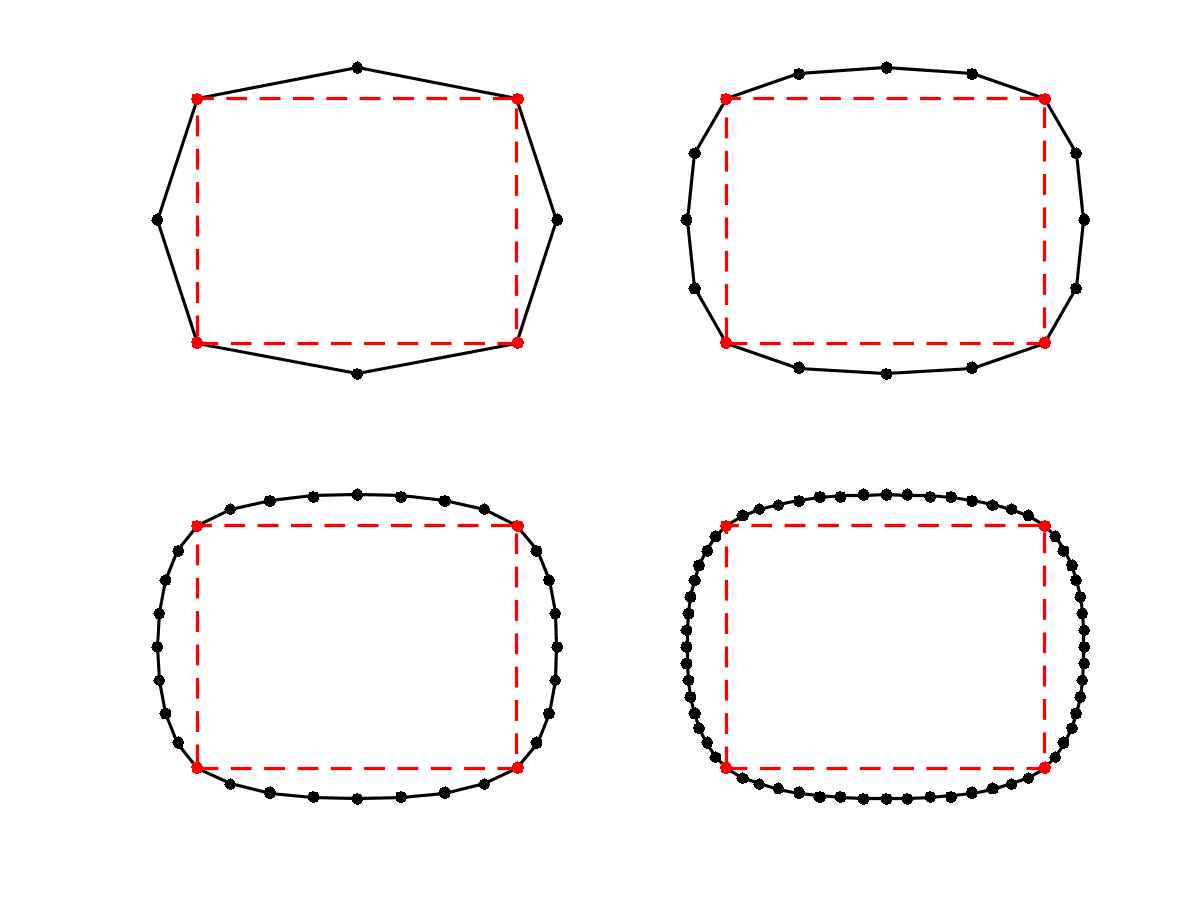In :
%% Insert your code here.


Exercise 7

Test the influence of $w$.

In :
exo7()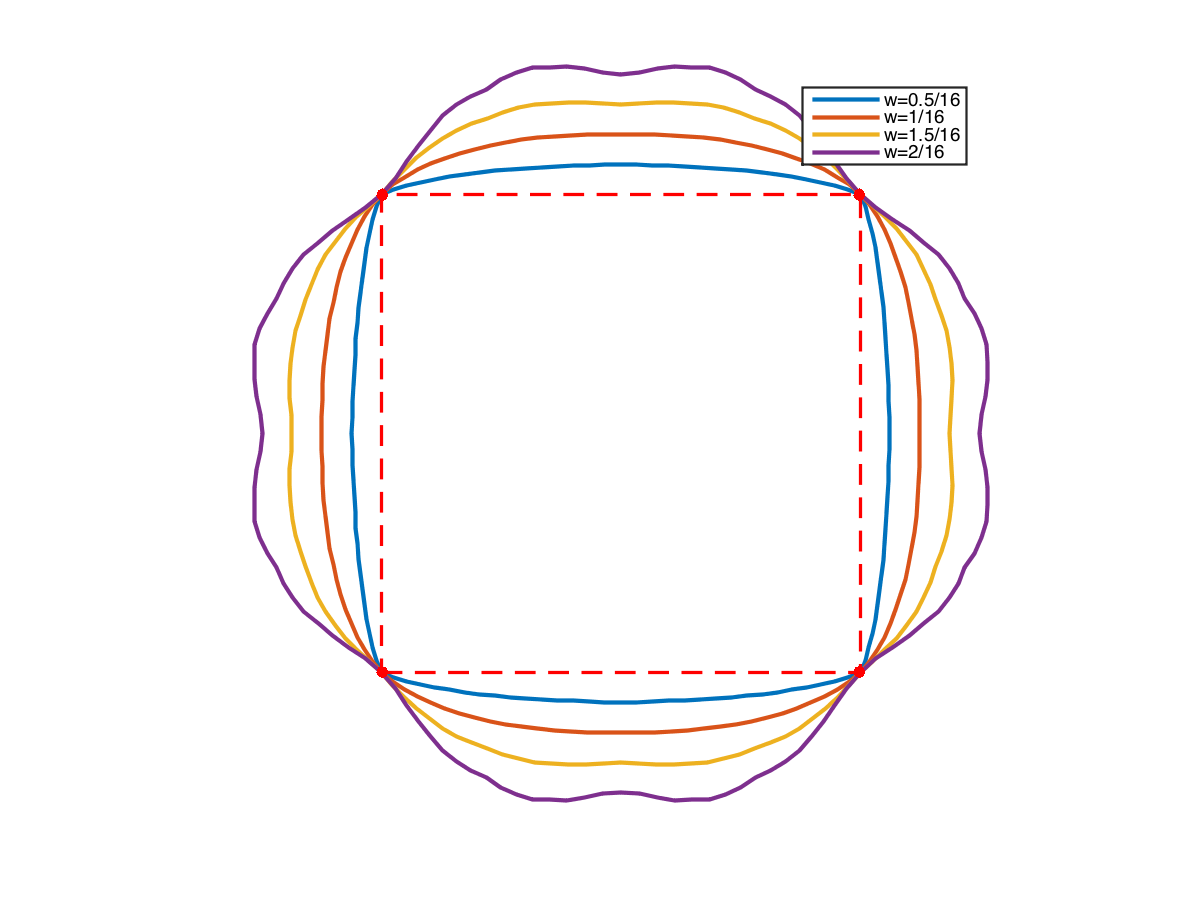In :
%% Insert your code here.


Exercise 8

Compare the result of the quadratic B-spline, cubic B-spline, and 4-points interpolating.

In :
exo8()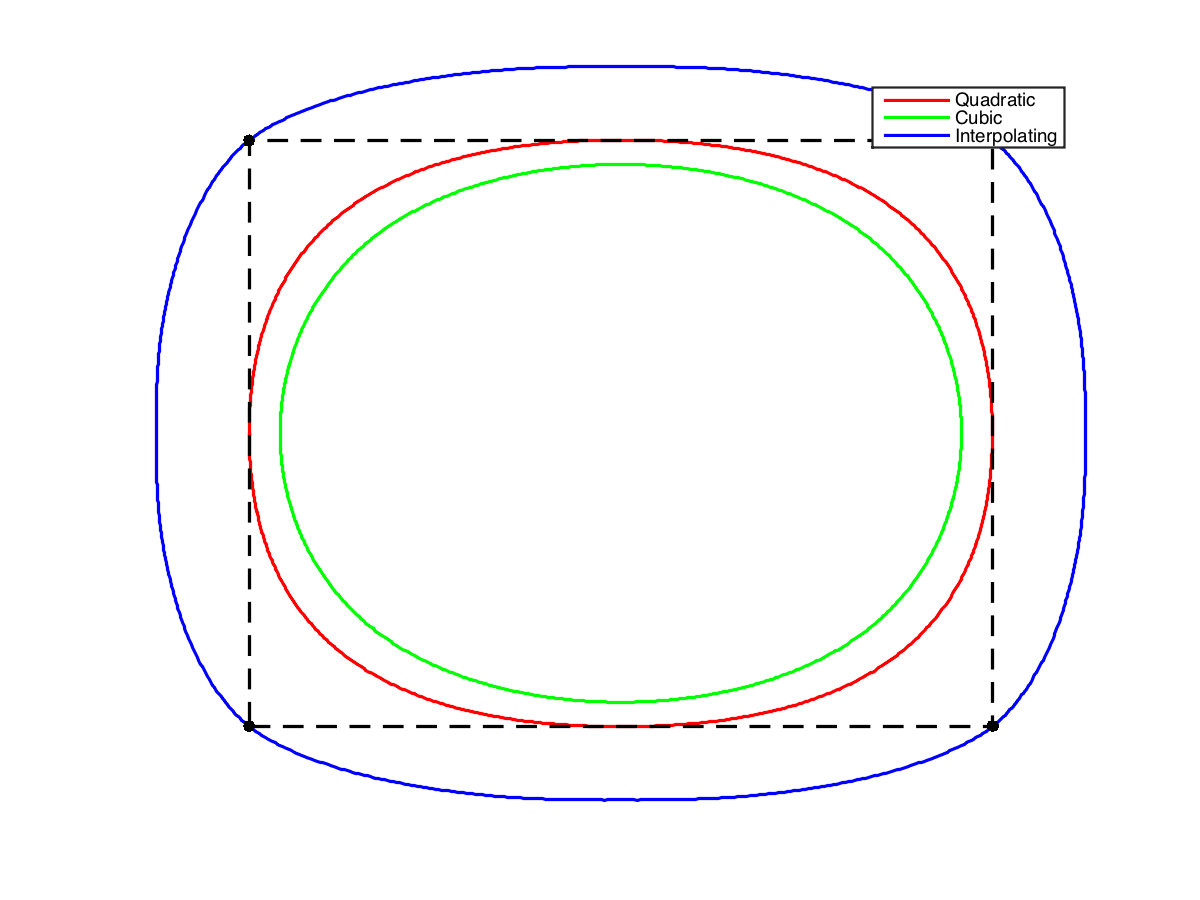In :
%% Insert your code here.


The 4-point scheme for $w=1/16$ is generalized to $2k$-point schemes of Deslauriers-Dubuc DeslDub89. This corresponds to computing a polynomial interpolation of degree $2k-1$, which generalizes the cubic interpolation. Using larger $k$ leads to smoother interpolation, at the price of a larger interpolation kernel.

We give here the odd coefficients of the filters.

In :
H = {   [0.5000 0.5000], ...
[-0.0625, 0.5625, 0.5625, -0.0625], ...
[0.0117, -0.0977, 0.5859, 0.5859, -0.0977, 0.0117], ...
[-0.0024, 0.0239, -0.1196, 0.5981, 0.5981, -0.1196, 0.0239, -0.0024] };
hdd = @(k)assign(assign(zeros(4*k-1,1),1:2:4*k-1,H{k}), 2*k, 1);


Exercise 9

Display the scaling function associated to these Deslauriers-Dubuc filters.

In :
exo9()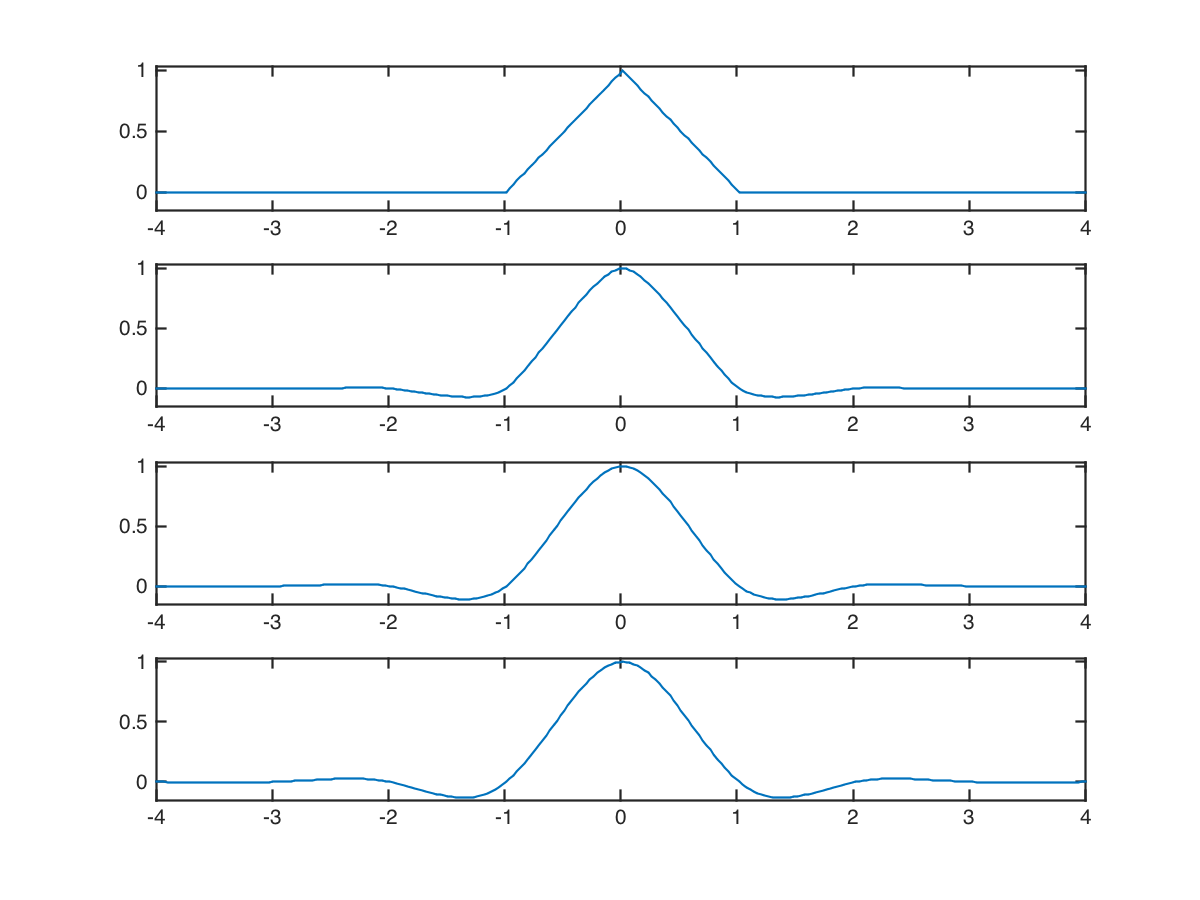In :
%% Insert your code here.


## Curve Approximation¶

Given an input, complicated curve $g : [0,1] \rightarrow \CC$, it is possible to approximate is by sampling the curve, and then subdividing it. It corresponds to a low pass filtering approximation.

Load an initial random curve, which is a high resolution curve $g$.

In :
options.bound = 'per';
n = 1024*2;
sigma = n/8;
F = perform_blurring(randn(n,1),sigma,options) + 1i*perform_blurring(randn(n,1),sigma,options);
F = rescale(real(F),.01,.99) + 1i * rescale(imag(F),.01,.99);


Display it.

In :
clf; myplot(F, 'k');
myaxis(0);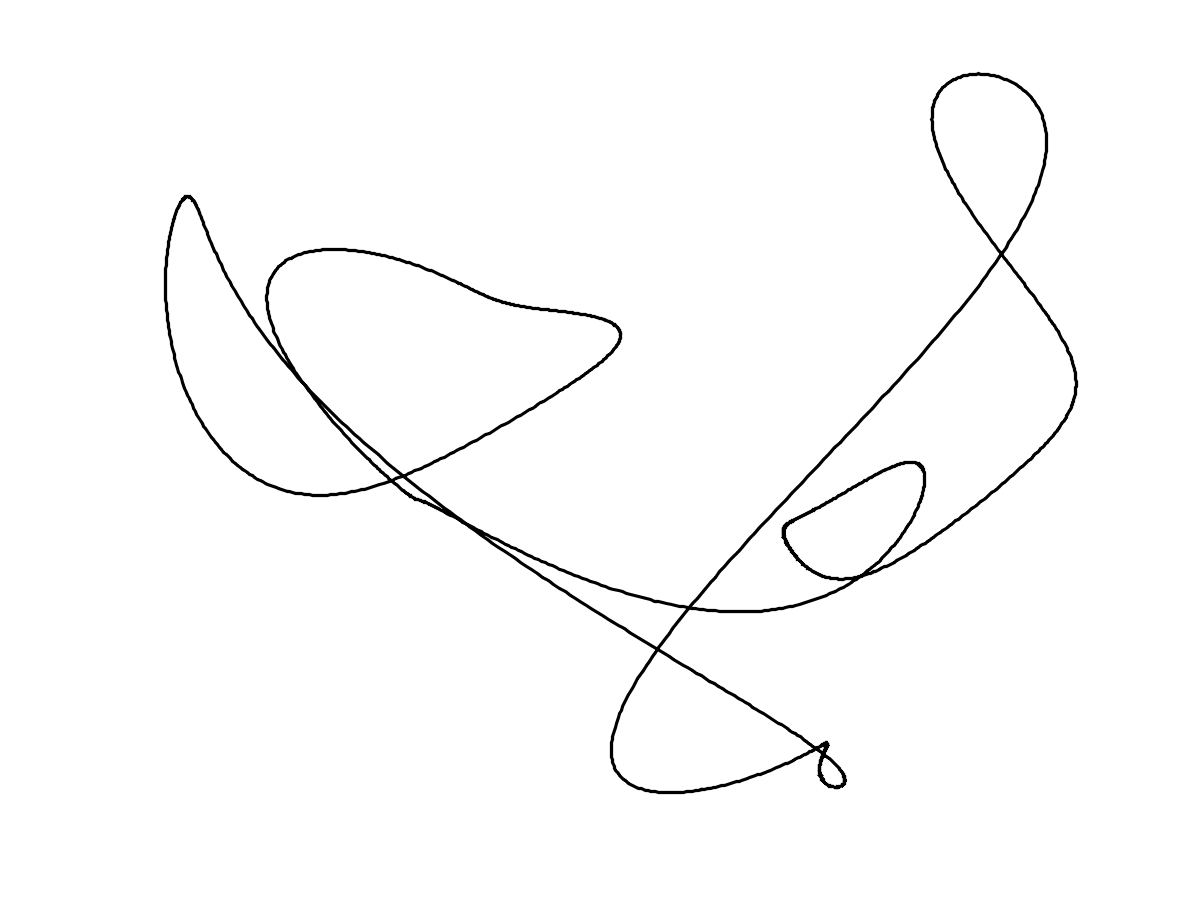In :
h = [-1, 0, 9, 1, 9, 0, -1]/16;
h((end+1)/2)=1;


Exercise 10

Perform an approximation $f$ of the curve using a uniform sampling with $N_0=20$ points.

In :
exo10()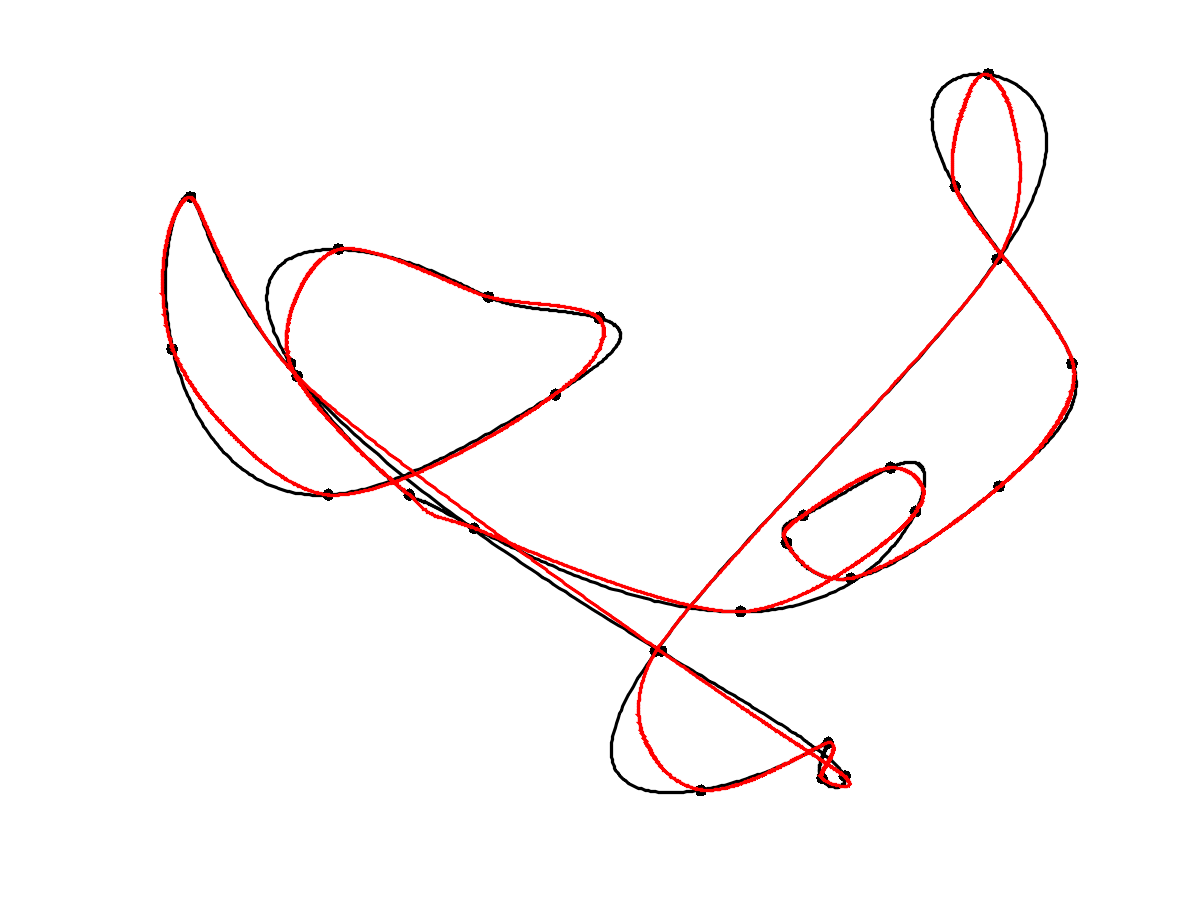In :
%% Insert your code here.


To quantify the quality of the approximation, we use an averaged Hausdorff distance. The distance between two sets of points $X$ and $Y$ is $$d(X,Y) = d_0(X,Y)+d_0(Y,X) \qwhereq d_0(X,Y)^2 = \frac{1}{\abs{X}} \sum_{x \in X} \umin{y \in Y} \norm{x-y}^2.$$

Compute the pairwise distances matrix $D_{i,j} = \norm{f_i-g_j}^2$ between points.

In :
dist = @(f,g)abs( repmat(f, [1 length(g)]) - repmat(transpose(g), [length(f) 1]) );


Compute the Hausdorff distance.

In :
hausdorff = @(f,g)sqrt( mean(min(dist(f,g)).^2) );
hausdorff = @(f,g)hausdorff(f,g) + hausdorff(g,f);


Exercise 11

Display the decay of the Hausdorff approximation error as the number $N_0$ of sampling points increases.

In :
exo11()